## Isothermal Reaction

exd13
Variables and parameter:

yi(t): chemical species, i=1,2,3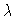: reaction rate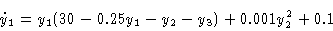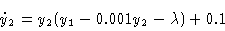There is a Hopf bifurcation at, and a sequence of period doubling bifurcations.

Figure 1
Bifurcation diagram, y1(0) versus.

Figure 2
Isothermal reaction, periodic orbit,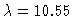, projection to (y1,y2) plane.

Figure 3
Isothermal reaction, periodic orbit,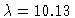, projection to (y1,y2) plane.

Figure 4
Isothermal reaction, chaotic orbit,, projection to (y1,y2) plane.

This Example
Figure 1
Figure 2
Figure 3
Figure 4